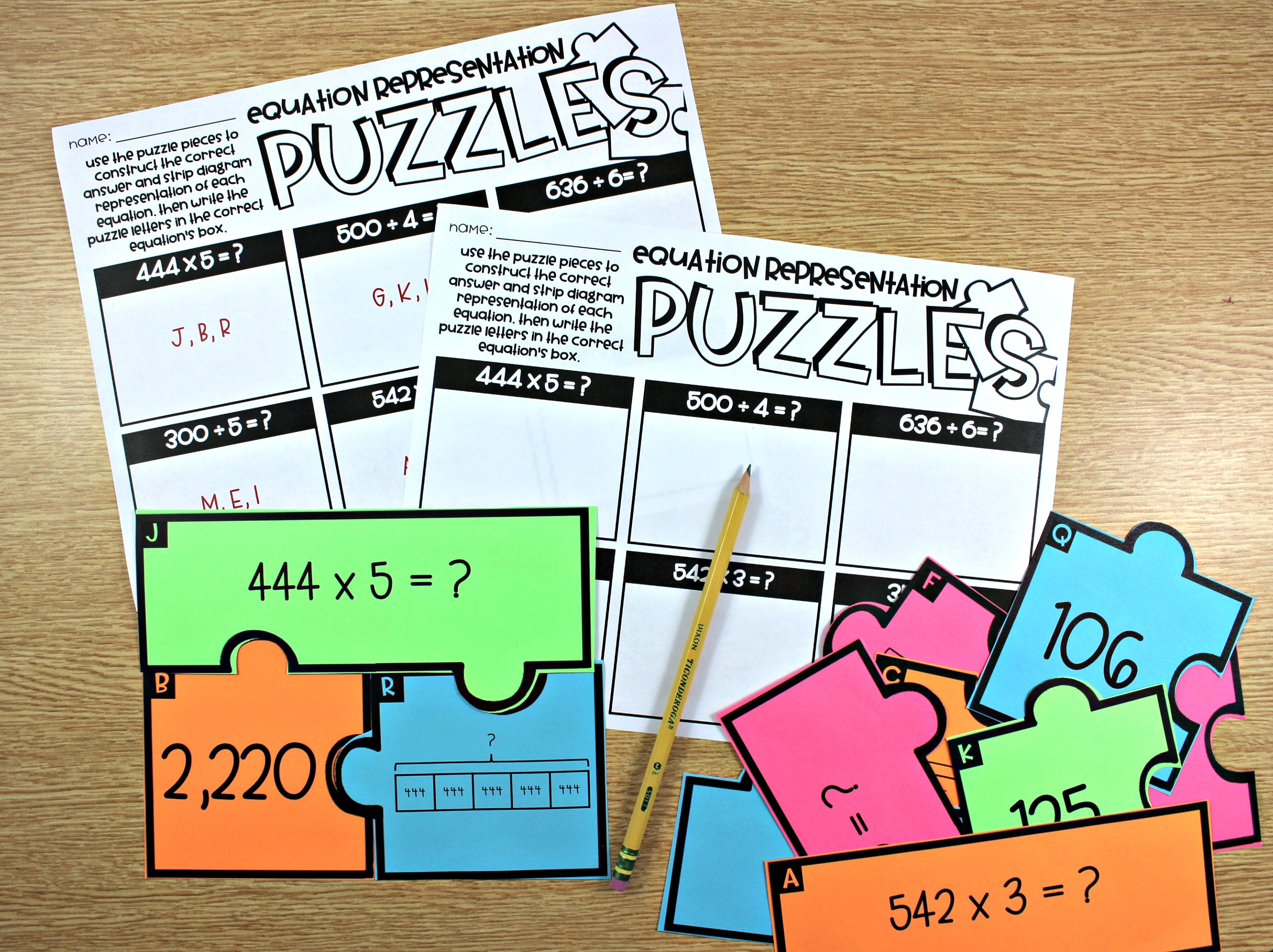The guided math series has grown!  Introducing….

I am thrilled to announce a new grade level of guided math is now available.  These lessons follow the components of guided math and give teacher everything needed to have a differentiated, standards-based math workshop.  The guided math bundle has all 9 guided math strands for fourth grade.

The units in the guided math series for 4th grade are:

• Place Value- to a Billion
• Multiplication and Division- Models and Algorithms
• Multiplication and Division- Strategies and Problem Solving
• Decimals- Investigating and Interpreting
• Fractions- Modeling and Representing
• Fractions- Comparing, Ordering, Adding, and Subtracting
• Measurement- Units and Conversions
• Geometry- Lines, Angles, and Shapes
• Data, Graphs, and Personal Finance

Each unit in the 4th grade guided math series contains:

• Problem of the day
• Guided math whole group mini-lessons with activities
• Guided math small group lessons with activities and differentiation
• Weekly quizzes
• Math notebook note taking with teacher keys
• Pre and Post Assessments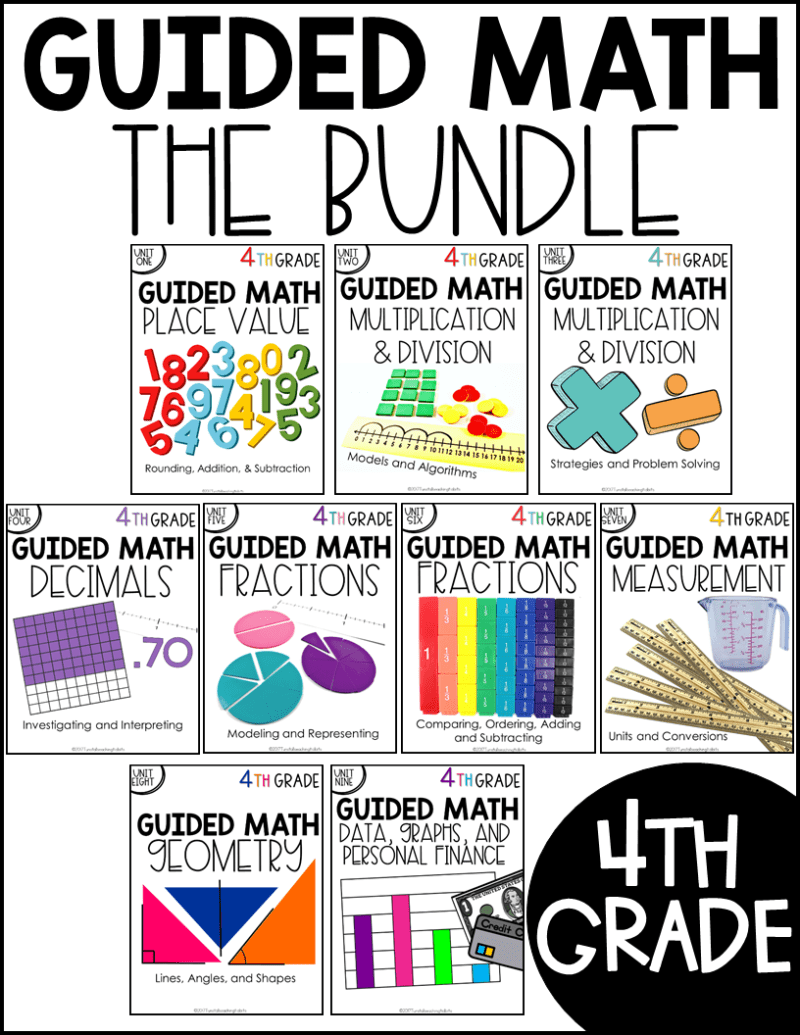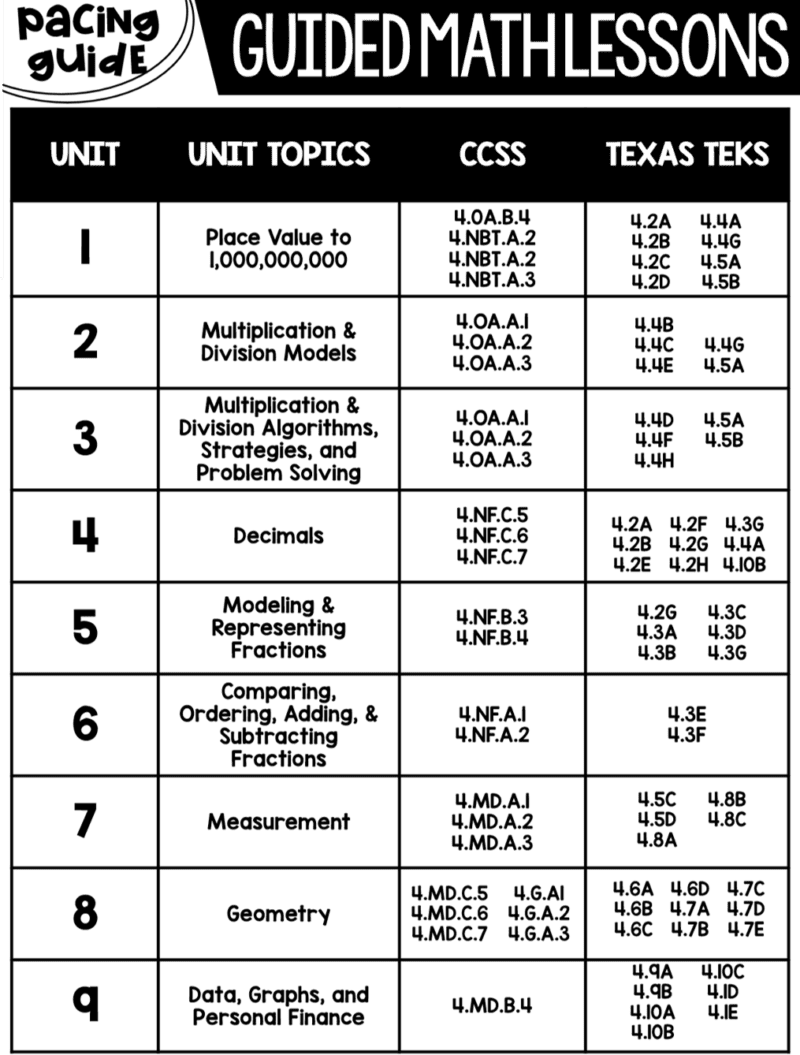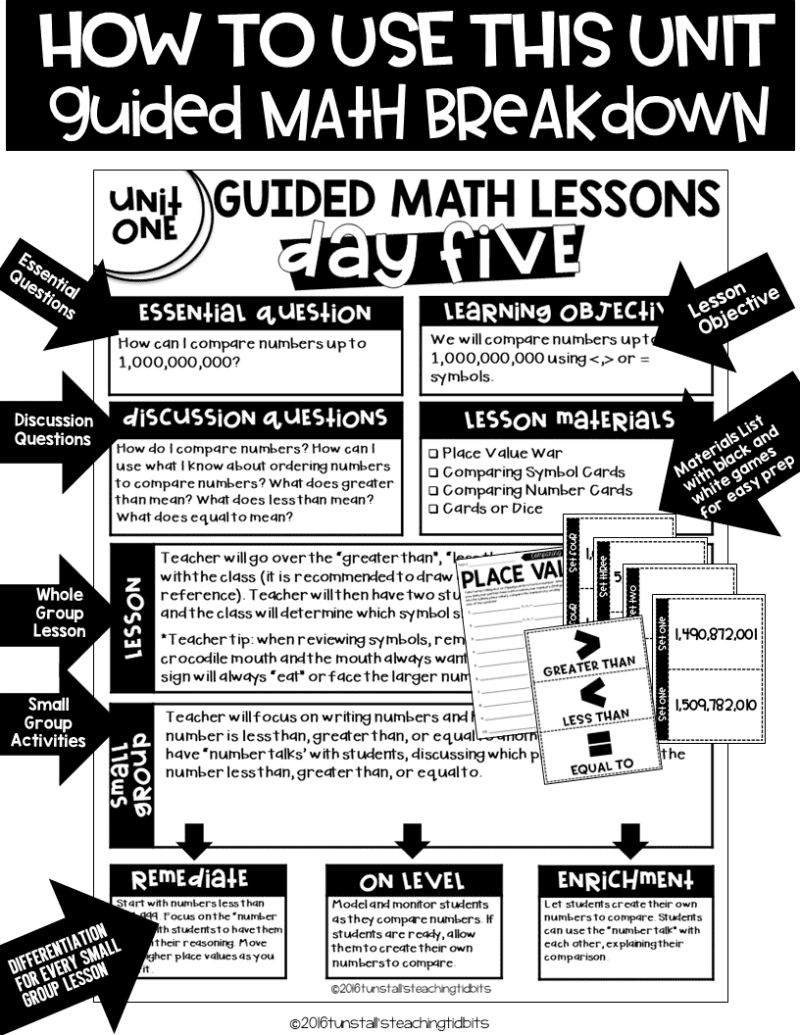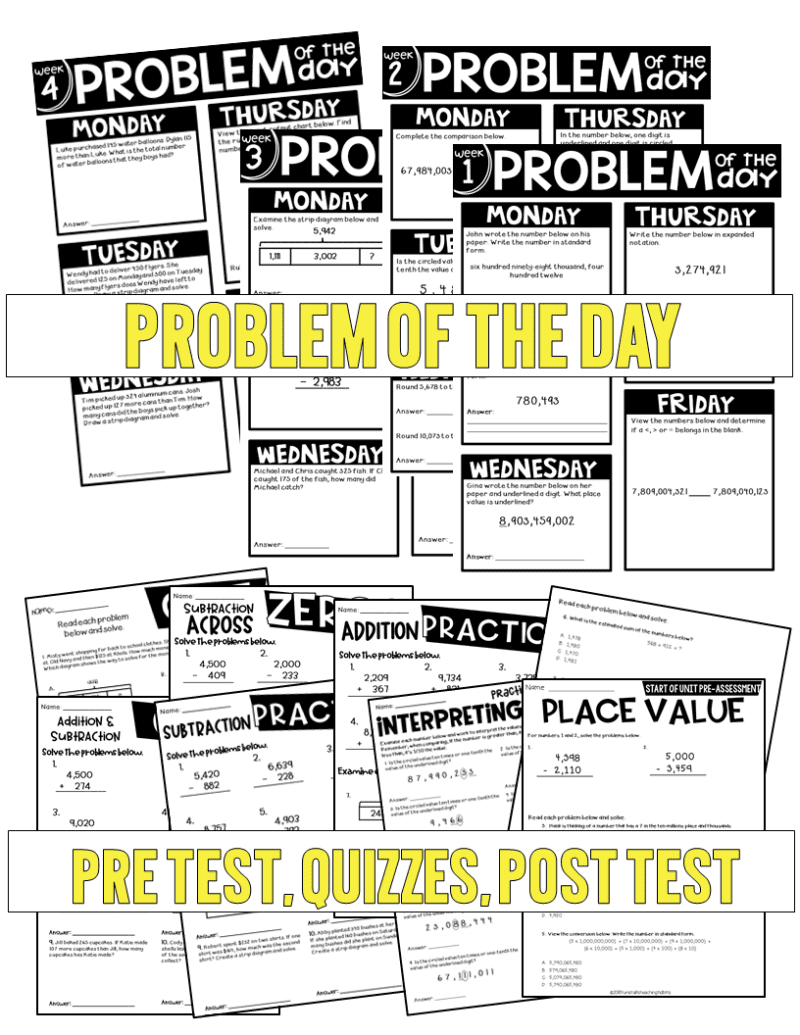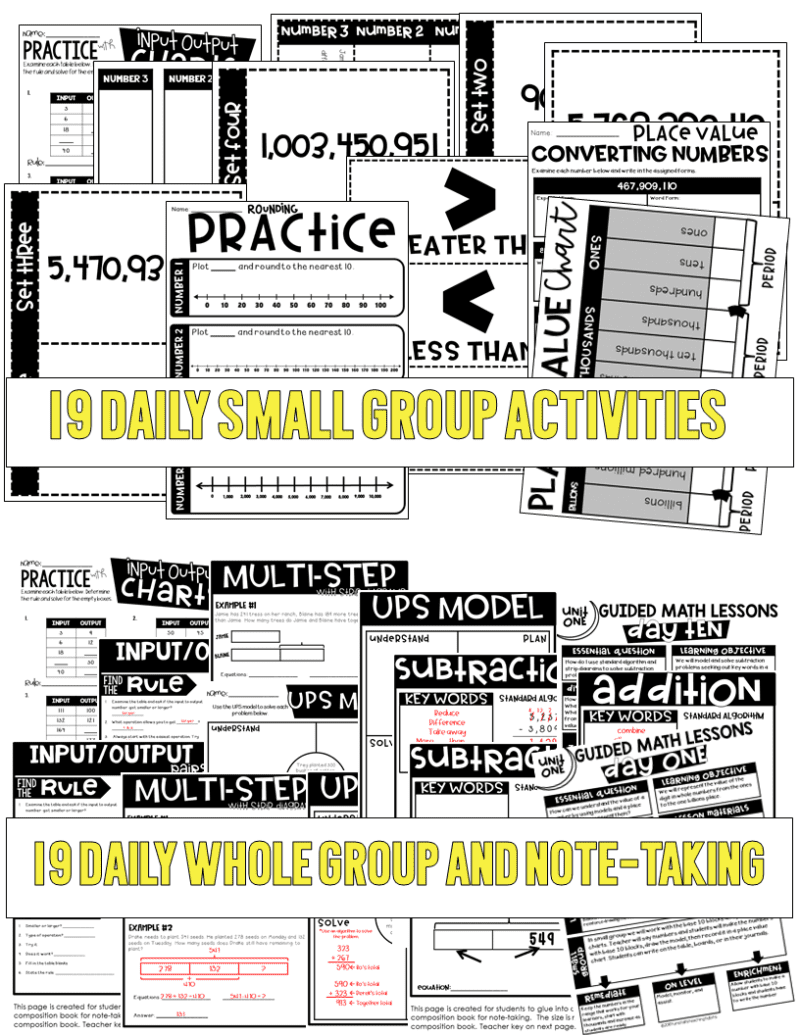Along with the guided math lessons for whole group and small group, I also have guided math centers for the each unit.  There are 10 activities to put in math rotations which provide students with time to apply the new skills being learned.  The math centers are there to provide reinforcement of the concepts.  Students can complete the activities in math rotations while teacher is able to teach a small group.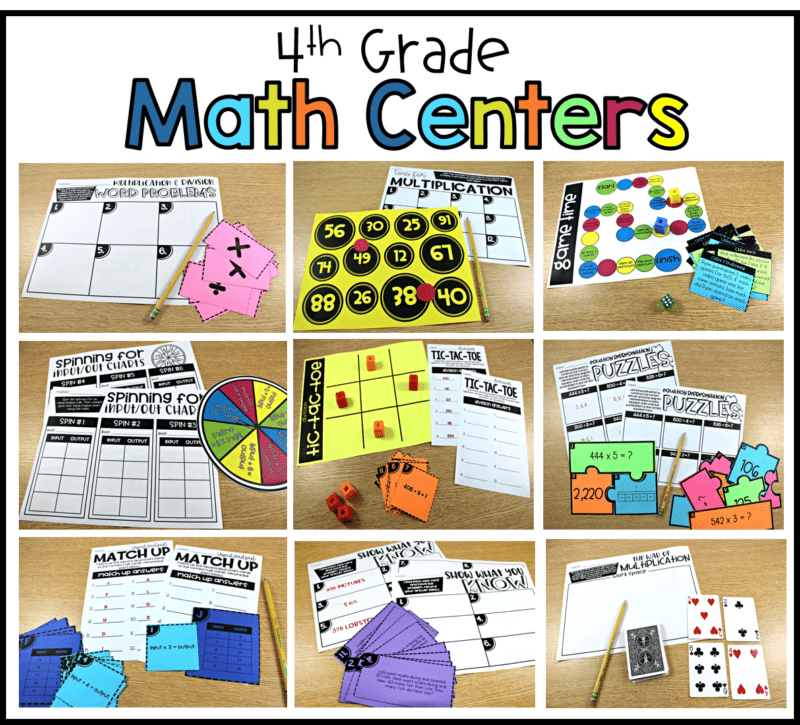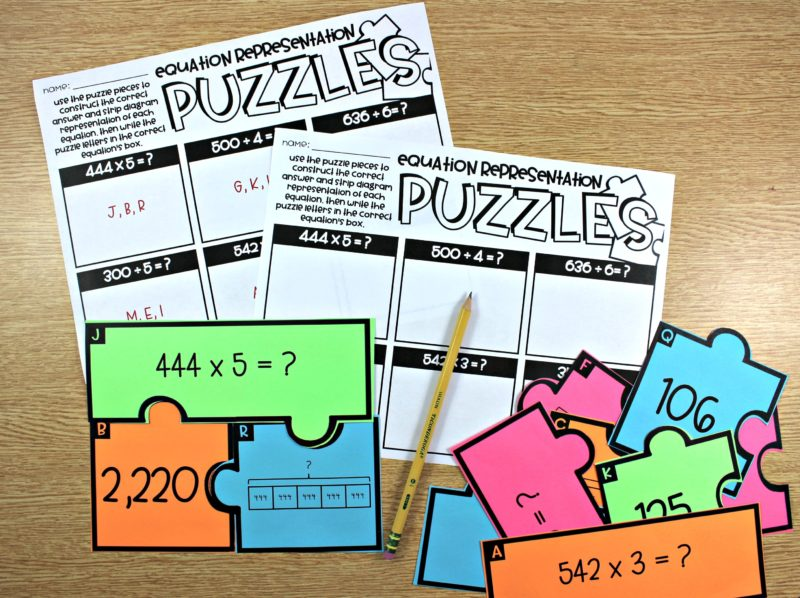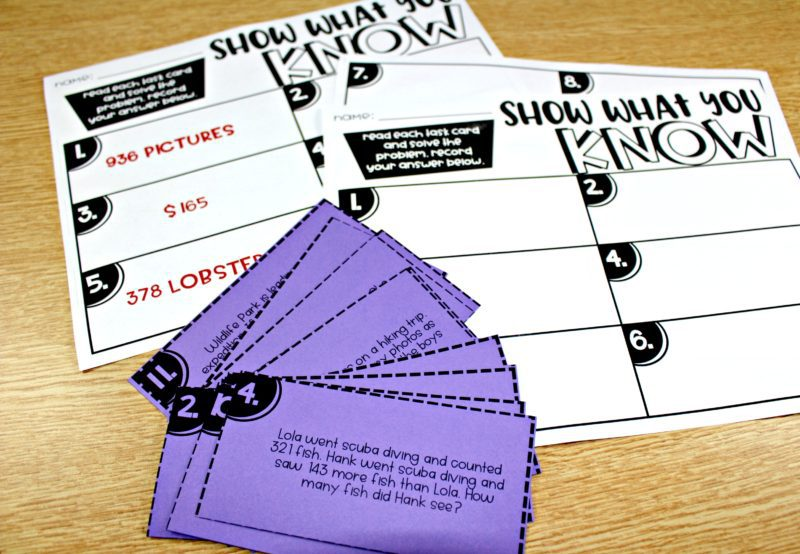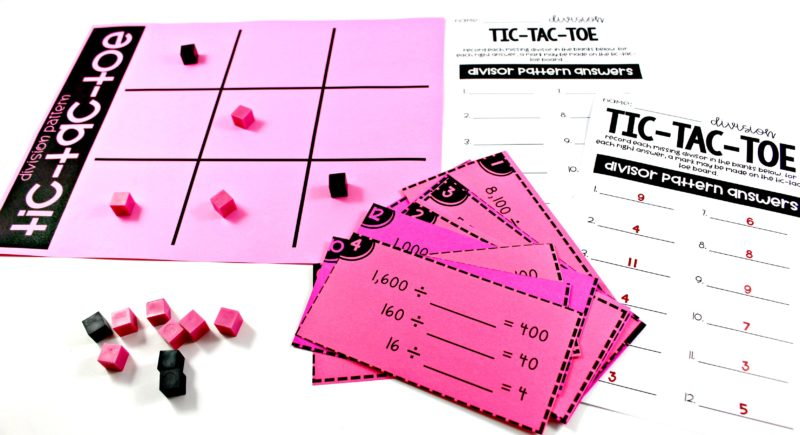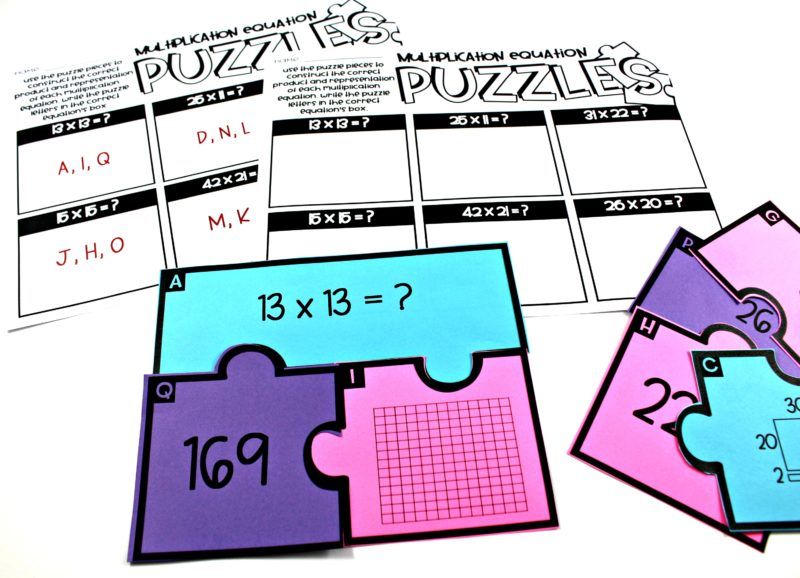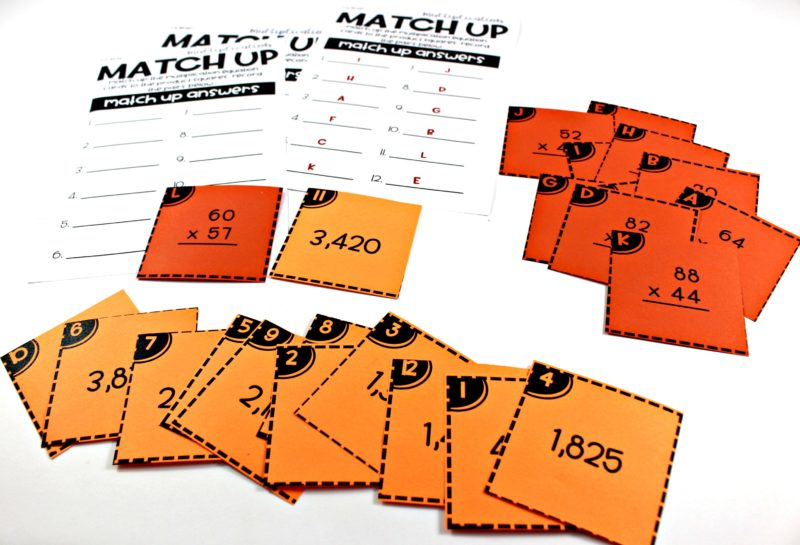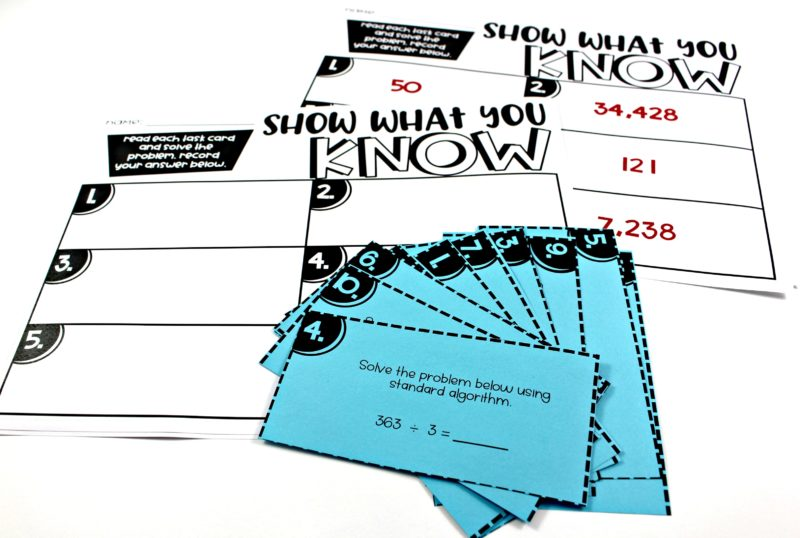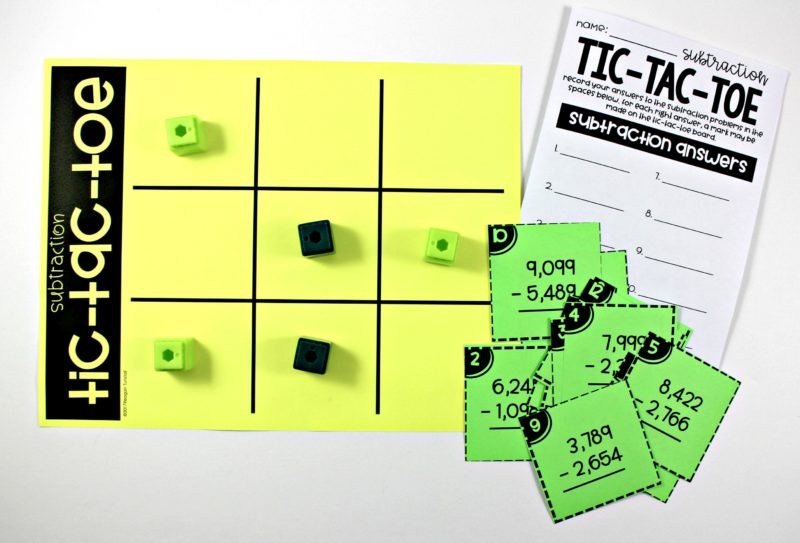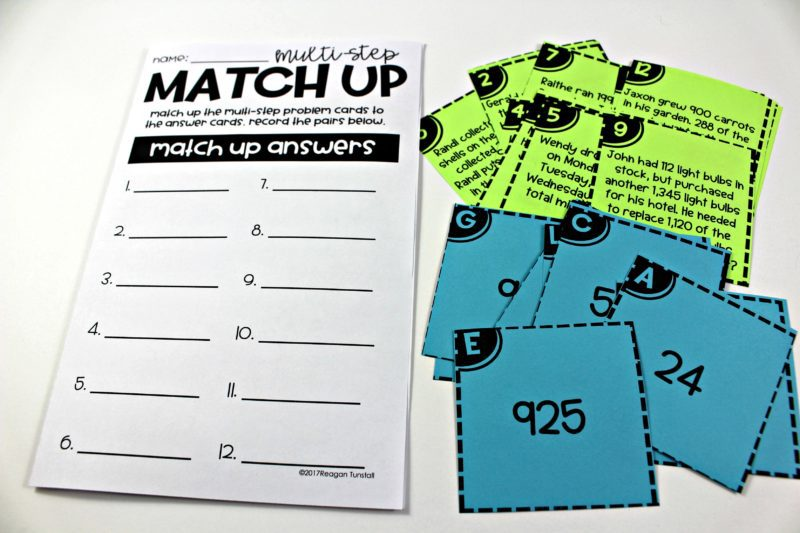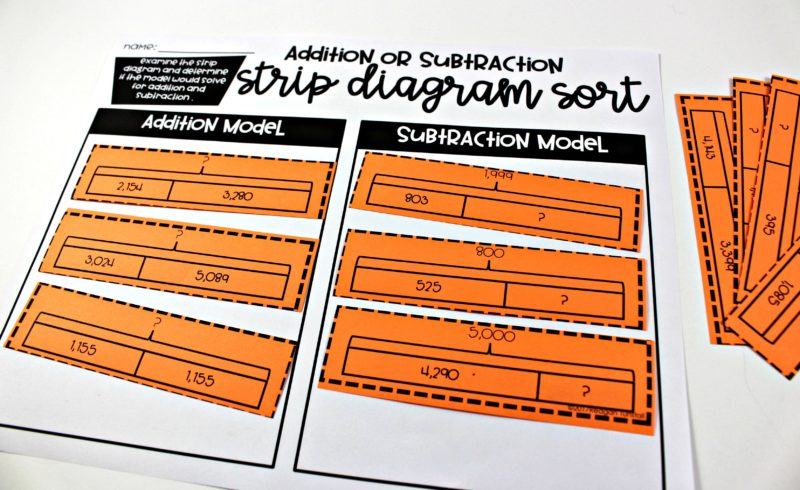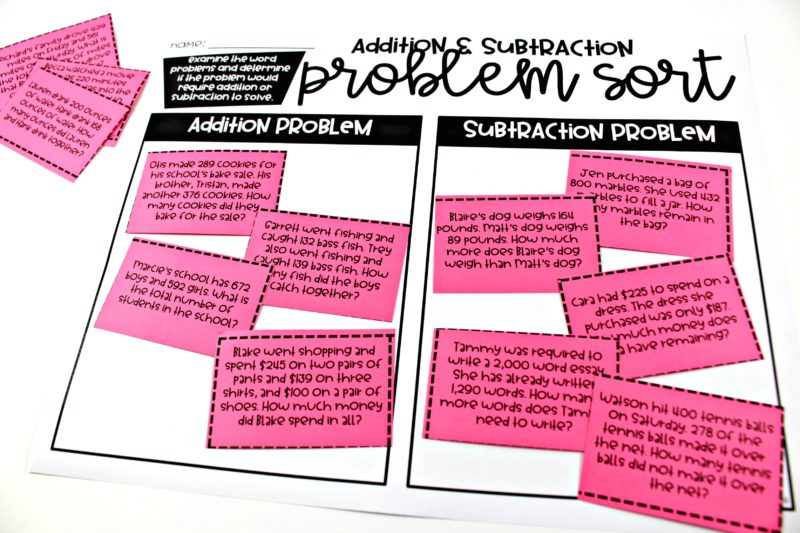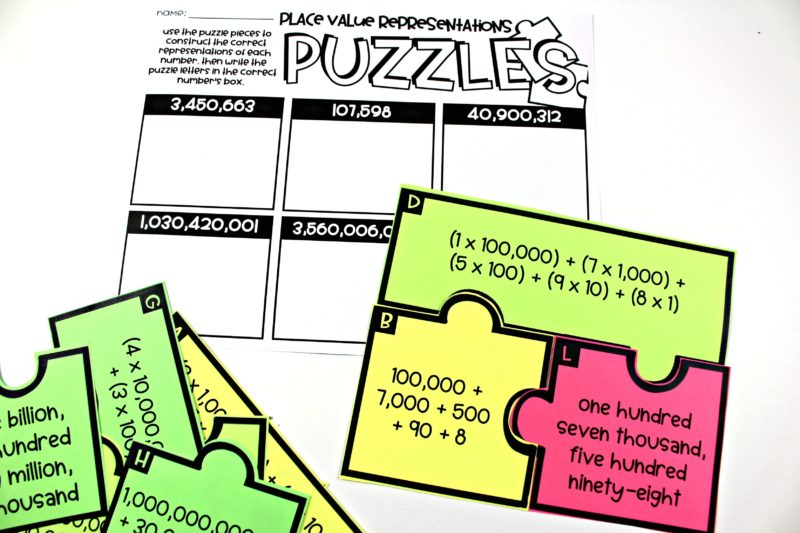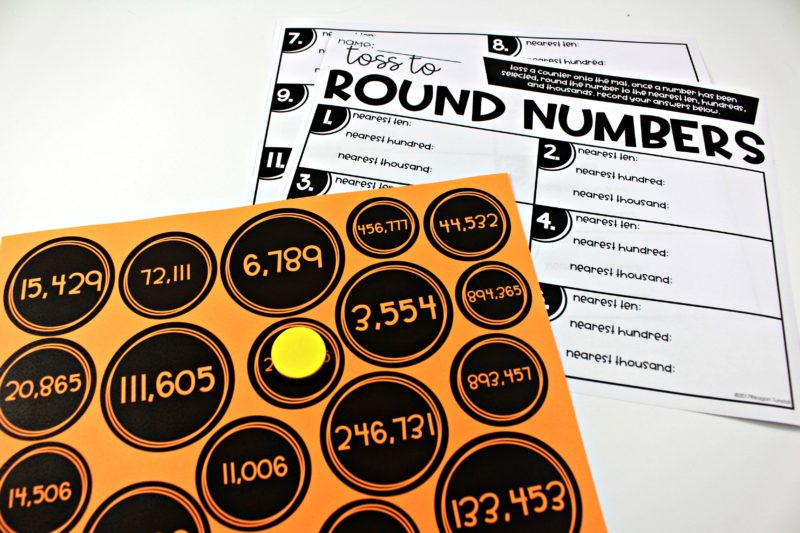Each math unit from the 4th grade guided math series has 10 math centers to give teacher the perfect station activities to reinforce the learning!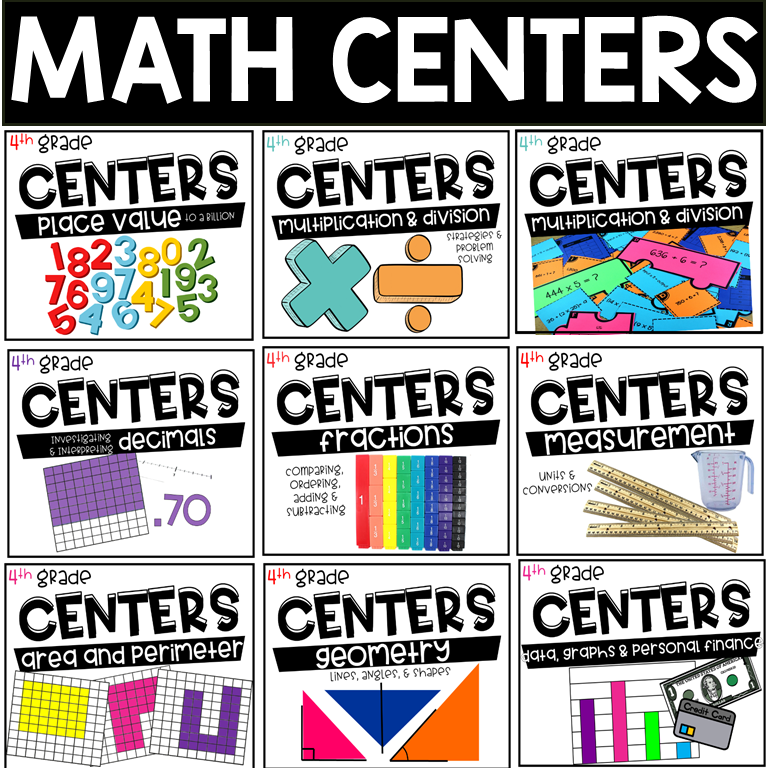## Similar Posts

1.Susan says:
2.Nicolle Macias says:
3.Leah says: